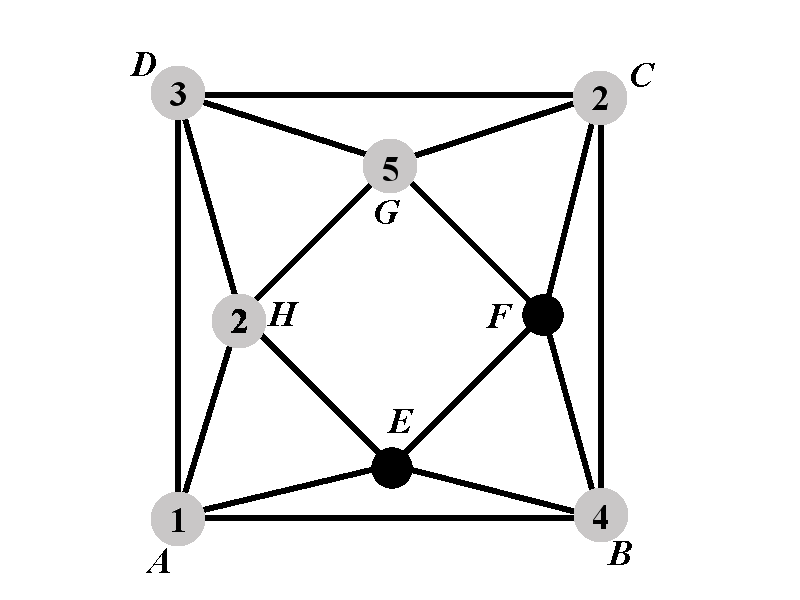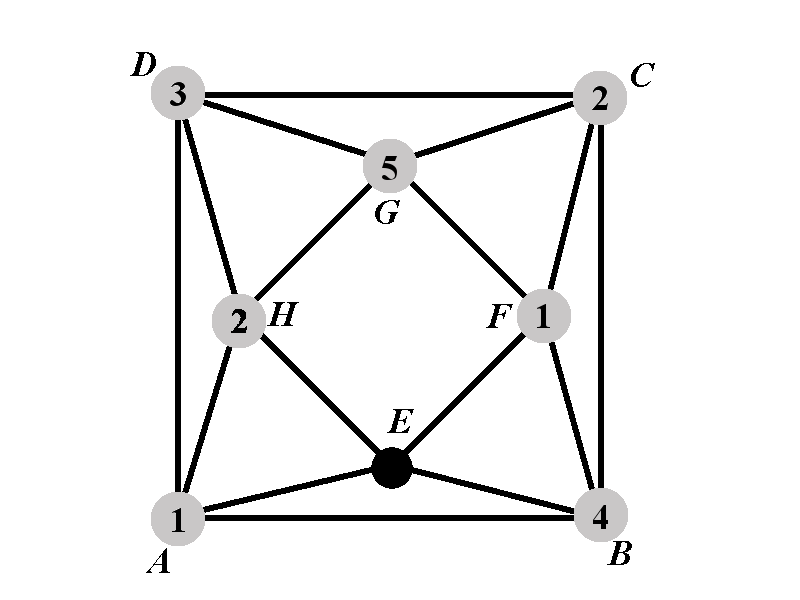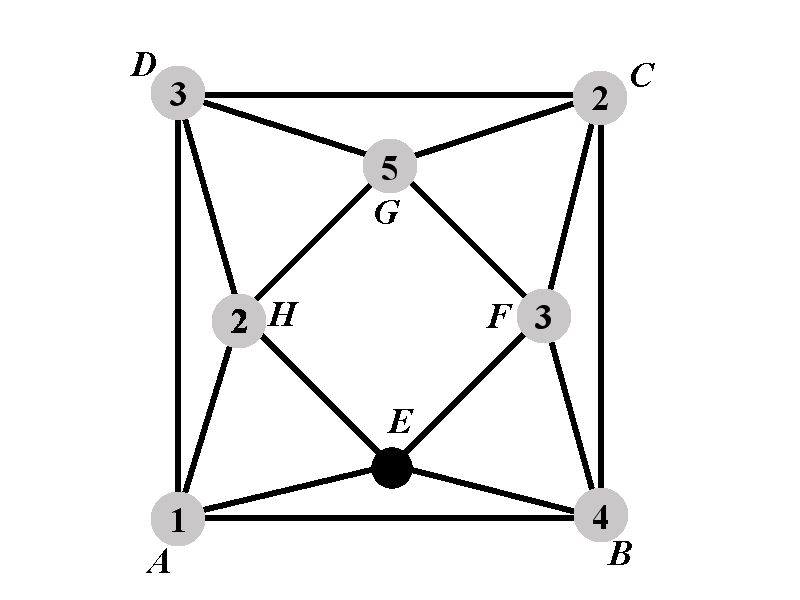Back to: Chromatic Polynomial Case $$2.2.1^{\circ}$$
##### Case 2.2.1.3: Calculation of $$P_{2.2.1.3^{\circ}}(k)$$The vertex $$F$$ cannot have colors $$2$$, $$4$$, and $$5$$. We will consider two sub-cases. In the sub-case 2.2.1.3.1 we count all the proper colorings of $$\Gamma$$ in which the vertex $$F$$ has color $$1$$. In the sub-case 2.2.1.3.2 we count all the proper colorings of $$\Gamma$$ in which the vertex $$F$$ does not have a color from $$\{1,2,4,5\}$$. In the sub-case 2.2.1.3.2 there are $$(k-4)$$ possible colors that can be assigned to $$F$$.

Let $$P_{2.2.1.3.1^{\circ}}(k)$$ be the number of proper colorings of $$\Gamma$$ in which the vertices $$A$$, $$B$$, $$C$$, $$D$$, $$F$$, $$G$$, and $$H$$ have the colors $$1$$, $$4$$, $$2$$, $$3$$, $$1$$, $$5$$, and $$2$$, respectively. Let $$P_{2.2.1.3.2^{\circ}}(k)$$ be the number of proper colorings of $$\Gamma$$ in which the vertices $$A$$, $$B$$, $$C$$, $$D$$, $$F$$, $$G$$, and $$H$$ have the colors $$1$$, $$4$$, $$2$$, $$3$$, $$3$$, $$5$$, and $$2$$, respectively. The following identity holds

$P_{2.2.1.3^{\circ}}(k)=P_{2.2.1.3.1^{\circ}}(k)+(k-4)P_{2.2.1.3.2^{\circ}}(k).$
##### Case 2.2.1.3.1: Calculation of $$P_{2.2.1.3.1^{\circ}}(k)$$It remains to count the number of ways in which the vertex $$E$$ can be colored. The vertex $$E$$ must not have any of the colors $$1$$, $$2$$, and $$4$$. That leaves $$k-3$$ available colors for the vertex $$E$$. Therefore $P_{2.2.1.3.1^{\circ}}(k)=k-3.$

##### Case 2.2.1.3.2: Calculation of $$P_{2.2.1.3.2^{\circ}}(k)$$The vertex $$E$$ must not have any of the colors $$1$$, $$2$$, $$3$$, and $$4$$. The number of available colors for $$E$$ is $$k-4$$. Therefore $P_{2.2.1.3.2^{\circ}}(k)=k-4.$

Thus we have \begin{eqnarray*}P_{2.2.1.3^{\circ}}(k)&=&P_{2.2.1.3.1^{\circ}}(k)+(k-4)P_{2.2.1.3.2^{\circ}}(k)\newline &=&k-3+(k-4)^2. \end{eqnarray*}